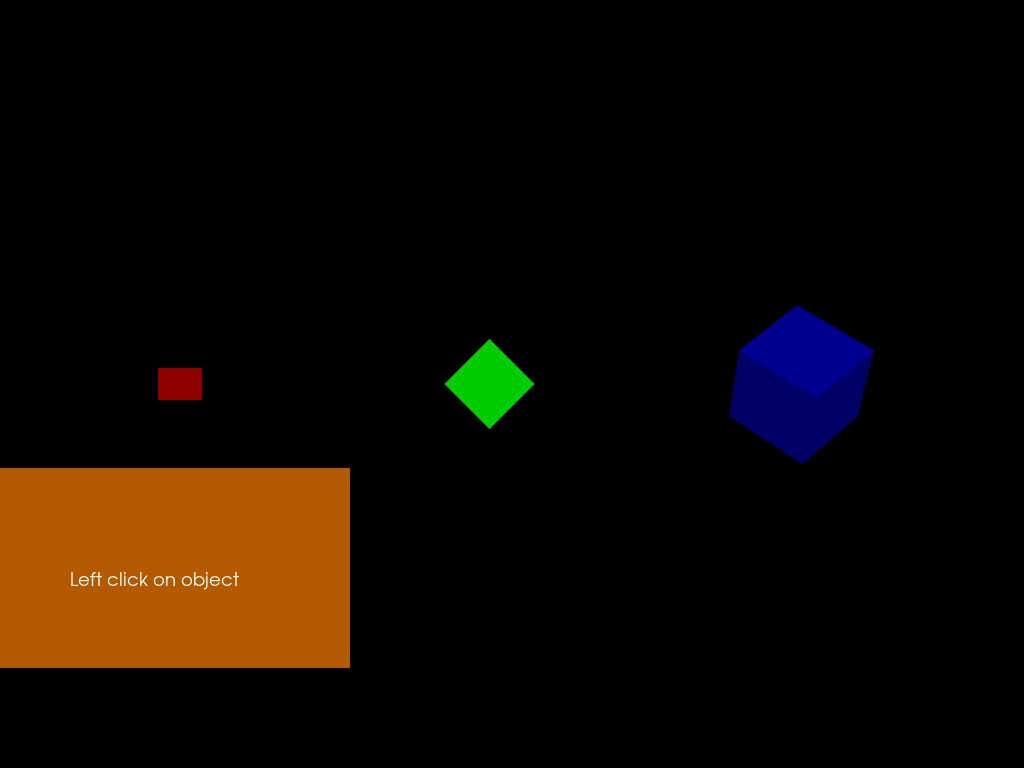# Simple picking¶

Here we present a tutorial showing how to interact with objects in the 3D world. All objects to be picked are part of a single actor. FURY likes to bundle objects in a few actors to reduce code and increase speed.

When the objects will be picked they will change size and color.

```import numpy as np
from fury import actor, window, ui, utils, pick

centers = 0.5 * np.array([[0, 0, 0], [100, 0, 0], [200, 0, 0.]])
colors = np.array([[0.8, 0, 0], [0, 0.8, 0], [0, 0, 0.8]])
radii = 0.1 * np.array([50, 100, 150.])

selected = np.zeros(3, dtype=np.bool)
```

Let’s create a panel to show what is picked

```panel = ui.Panel2D(size=(400, 200), color=(1, .5, .0), align="right")
panel.center = (150, 200)

text_block = ui.TextBlock2D(text="Left click on object \n")
```

Build scene and add an actor with many objects.

```scene = window.Scene()

label_actor = actor.label(text='Test')
```

This actor is made with 3 cubes of different orientation

```directions = np.array([[np.sqrt(2)/2, 0, np.sqrt(2)/2],
[np.sqrt(2)/2, np.sqrt(2)/2, 0],
[0, np.sqrt(2)/2, np.sqrt(2)/2]])
fury_actor = actor.cube(centers, directions, colors, heights=radii)
```

Out:

```/Users/koudoro/Software/fury/docs/tutorials/01_introductory/viz_picking.py:46: ArgsDeprecationWarning: "heights" was deprecated

* deprecated from version: 0.6
* Will raise <class 'fury.deprecator.ExpiredDeprecationError'> as of version: 0.8
fury_actor = actor.cube(centers, directions, colors, heights=radii)
```

Access the memory of the vertices of all the cubes

```vertices = utils.vertices_from_actor(fury_actor)
num_vertices = vertices.shape
num_objects = centers.shape
```

Access the memory of the colors of all the cubes

```vcolors = utils.colors_from_actor(fury_actor, 'colors')
```

Adding an actor showing the axes of the world coordinates

```ax = actor.axes(scale=(10, 10, 10))

scene.reset_camera()
```

Create the Picking manager

```pickm = pick.PickingManager()
```

Time to make the callback which will be called when we pick an object

```def left_click_callback(obj, event):

# Get the event position on display and pick

event_pos = pickm.event_position(showm.iren)
picked_info = pickm.pick(event_pos, showm.scene)

vertex_index = picked_info['vertex']

# Calculate the objects index

object_index = np.int(np.floor((vertex_index / num_vertices) *
num_objects))

# Find how many vertices correspond to each object
sec = np.int(num_vertices / num_objects)

if not selected[object_index]:
scale = 6/5
color_add = np.array([30, 30, 30], dtype='uint8')
selected[object_index] = True
else:
scale = 5/6
color_add = np.array([-30, -30, -30], dtype='uint8')
selected[object_index] = False

# Update vertices positions
vertices[object_index * sec: object_index * sec + sec] = scale * \
(vertices[object_index * sec: object_index * sec + sec] -
centers[object_index]) + centers[object_index]

# Update colors
vcolors[object_index * sec: object_index * sec + sec] += color_add

# Tell actor that memory is modified
utils.update_actor(fury_actor)

face_index = picked_info['face']

# Show some info
text = 'Object ' + str(object_index) + '\n'
text += 'Vertex ID ' + str(vertex_index) + '\n'
text += 'Face ID ' + str(face_index) + '\n'
text += 'World pos ' + str(np.round(picked_info['xyz'], 2)) + '\n'
text += 'Actor ID ' + str(id(picked_info['actor']))
text_block.message = text
showm.render()
```

Bind the callback to the actor

```fury_actor.AddObserver('LeftButtonPressEvent', left_click_callback, 1)
```

Make the window appear

```showm = window.ShowManager(scene, size=(1024, 768), order_transparent=True)
showm.initialize()
```

Change interactive to True to start interacting with the scene

```interactive = False

if interactive:

showm.start()
```

Save the current framebuffer in a PNG file

```window.record(showm.scene, size=(1024, 768), out_path="viz_picking.png")
```Total running time of the script: ( 0 minutes 0.161 seconds)

Gallery generated by Sphinx-Gallery